Home > CC4 > Chapter 7 > Lesson 7.1.4 > Problem7-48

7-48.
1. For parts (a) and (b), write a recursive equation using an notation for each sequence. For parts (c) and (d), write an explicit equation using t(n) notation for each sequence. (For a reminder about sequence notation, see the Math Notes box in Lesson 5.3.2.) Homework Help ✎

1. 108, 120, 132, …

2.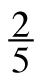,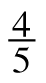,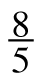, …

3. 3741, 3702, 3663, …

4. 117, 23.4, 4.68, …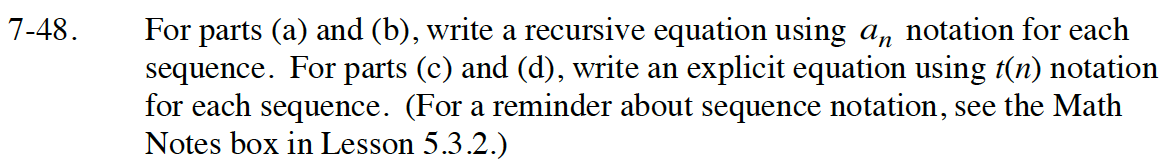Read Math Notes box in Lesson 5.3.3 on equations for sequences.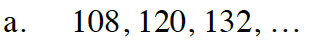a1 = 108
an+1 = an+12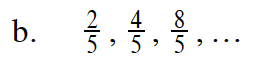$a_1=\frac{2}{5}$

a(n + 1) = 2an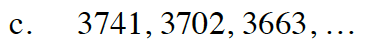Is the sequence arithmetic or geometric?
Remember to write an explicit equation.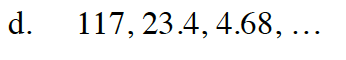Is the sequence arithmetic or geometric?
Remember to write an explicit equation.